#### You may also like### Time of Birth

A woman was born in a year that was a square number, lived a square number of years and died in a year that was also a square number. When was she born?### Sending a Parcel

What is the greatest volume you can get for a rectangular (cuboid) parcel if the maximum combined length and girth are 2 metres?### Litov's Mean Value Theorem

Start with two numbers and generate a sequence where the next number is the mean of the last two numbers...

# Cola Can

##### Age 11 to 14Challenge Level

There is a Stage 5 topic called Differentiation (part of Calculus) which makes these sorts of problems rather easy.

At Stage 4 the challenge is to handle the algebra well (the formulae that calculate, height or diameter and then surface area) and to use a trial and improvement method to get as close as we wish to the answer.

Using a spreadsheet takes a lot of the labour out of trial and improvement, and also helpfully makes an automatic table of results.

We had lots of solutions using Stage 4 mathematics, but well done in particular to Oliver from Olchfa, Michael from Homestead High, Sam and Rachel both from Millais School, and David from Gordonstoun .

The volume of a cylinder is found from the circular cross-section multiplied by the distance over which it continues, and here the volume has to be 330 ml.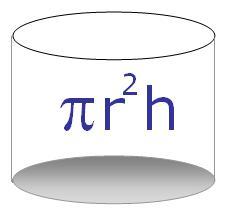So when the can's diameter is 6 cm (r=3) it's height (h) is 11.67 cm.

And when it's height is 10 cm, the can's diameter has to be 6.48 cm

We notice that if the volume is fixed then whether we know the can's diameter or it's height, the other of these two will be easy enough to calculate using :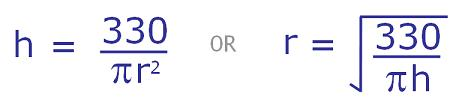So whichever we already have, either r or h, we can find the other and then use both in the surface area formula.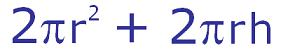That's two circles, top and bottom, plus a rectangle (can circumference by can height - like peeling the label off a tin).

We are now going to work systematically to find the diameter and height of the can (volume 330 ml) which has the least surface area.

We could start with a radius of, say, 1 cm and find the height which makes a volume of 330 ml, then use both those r and h values to calculate the surface area for that can.

We could then step up the radius to 2 cm and repeat the calculations.

In fact we could continue to increase the radius and make up a table of the surface area results each time.

Alternatively we could use the same method but start with a value for height instead, and increase that.

Once we see how the surface area changes with radius (or height) in a general way we can refine our choice of an r or h value until, by trial and improvement, we get as near as we please to the lowest value for the surface area.

Here's the table Sam made :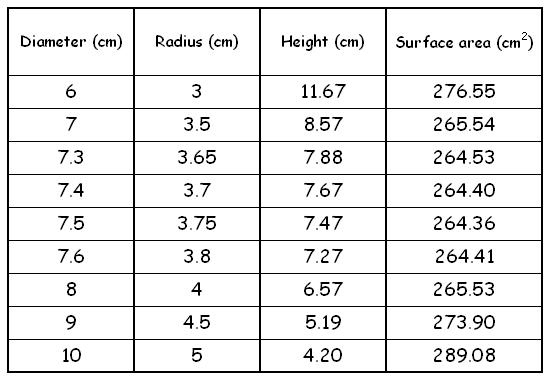And here's a graph used by Michael showing an interesting and non-symmetric curve for the changing surface area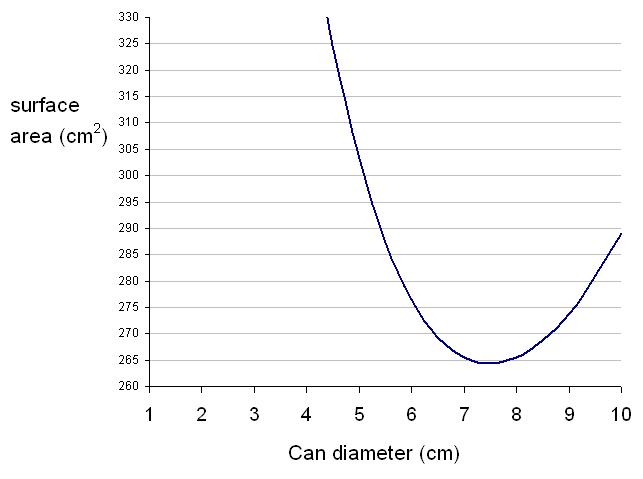For those who like to see nice things done with spreadsheets in mathematics here's a link to an Excel file that does all that calculation in an instant : Cola Can

Very well done to everyone who engaged with this problem, we were really pleased to see how popular it had been. Why not look at the Funnel problem - trying to use the least amount of plastic to make a funnel .